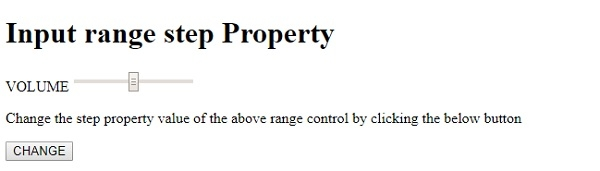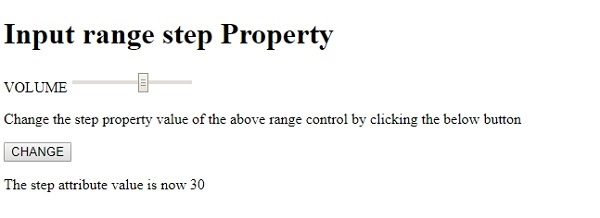HTML DOM Input Range step property

HTMLWeb DevelopmentFront End Technology

The HTML DOM Input Range step property is used for setting or returning the slider control step attribute value. It specifies how much the slider will move on each movement. By using the max and min attribute with the step property, we can define a range of legal values.

Syntax

Following is the syntax for −

Setting the step property −

rangeObject.step=number

Here, number specifies the slider control movement size. The default value for this is 1.

Example

Let us look at an example for the Input Range step property −

Live Demo

<!DOCTYPE html>
<html>
<body>
<h1>Input range step Property</h1>
<form>
VOLUME <input type="range" id="RANGE1" name="VOL">
</form>
<p>Change the step property value of the above range control by clicking the below button</p>
<button type="button" onclick="changeStep()">CHANGE</button>
<p id="Sample"></p>
<script>
function changeStep() {
document.getElementById("RANGE1").step ="30" ;
document.getElementById("Sample").innerHTML = "The step attribute value is now 30";
}
</script>
</body>
</html>

Output

This will produce the following output −On clicking the CHANGE button −In the above example −

We have created an input field contained inside a form having type=“range”, id=“RANGE1” ,name=“VOL”. Its step property value is by default set to 1 −

<form>
VOLUME <input type="range" id="RANGE1" name="VOL">
<form>

We have then created a button CHANGE that will execute the changeStep() method on being clicked by the user −

<button type="button" onclick="changeStep()">CHANGE</button>

The changeStep() method uses the getElementById() method to get the input field with type range by passing the id “RANGE1” to it. After getting the element we set its step property to 30. This will increase how much the slider will move in one movement. Since the min value is 0 and max value is 100 by default so the slider will move to only three places now −

function changeStep() {
document.getElementById("RANGE1").step ="30" ;
document.getElementById("Sample").innerHTML = "The step attribute value is now 30";
}